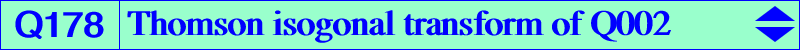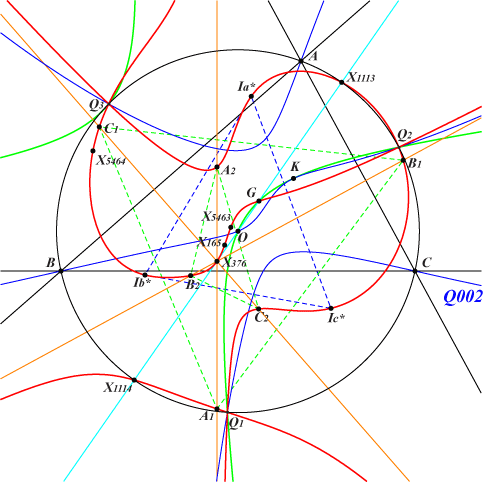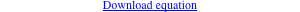too complicated to be written here. Click on the link to download a text file.X(2), X(165), X(376), X(1113), X(1114), X(5463), X(5464), X(11228) vertices of the Thomson triangle T = Q1Q2Q3 O1, O2 foci of the inconic of T with center O S1, S2, S3 : its contacts with the sidelines of T other points belowQ178 is the isogonal transform of the Euler-Morley quartic Q002 with respect to the Thomson triangle Q1Q2Q3. Q178 is a circular quintic with singular focus G. It has three real asymptotes perpendicular at X(376) to the sidelines of ABC. The remaining points A1, B1, C1 and A2, B2, C2 of Q178 on these asymptotes are the Thomson isogonal conjugates of the feet of the bisectors of ABC. Q178 also contains the conjugates of the in/excenters of ABC, namely X(5463) and Ia*, Ib*, Ic*. *** Properties of these triangles • Ia* = a (3 a^2+2 a b-b^2+2 a c+2 b c-c^2) : b (a^2-2 a b-3 b^2+2 a c+2 b c+c^2) : c (a^2+2 a b+b^2-2 a c+2 b c-3 c^2). Ia* = Thomson isogonal conjugate of the excenter Ia of ABC. Ia*Ib*Ic* is homothetic to the 1st circumperp triangle (circum-anticevian triangle of X1) under h(X1, 2/3). It is also homothetic to the intouch triangle at X(3601) and to the excentral and 2nd circumperp triangles at X(1). It is perspective to the cevian triangle of every point on a circum-cubic passing through X(7), X(21), X(57), X(63), X(86), X(16948). It is orthologic to the cevian triangle of every point of K034 and to the anticevian triangle of every point of K345. In both cases, the centers of orthology lie on K033 and K758. It is orthologic and parallelogic to the circumcevian triangle of X(36) hence inversely similar to this triangle. *** • A1 = 3 a^4-2 a^2 b^2-b^4+4 a^2 b c-2 a^2 c^2+2 b^2 c^2-c^4 : 2 b (-2 a^2 b+2 b^3-a^2 c-b^2 c-2 b c^2+c^3) : 2 c (-a^2 b+b^3-2 a^2 c-2 b^2 c-b c^2+2 c^3). A1 = Thomson isogonal conjugate of 0 : b : -c, the trace of the antiorthic axis on BC. A1, B1, C1 obviously lie on the ellipse with center X(3576) passing through X(104), Q1, Q2, Q3 which is the Thomson isogonal transform of the antiorthic axis of ABC. A1B1C1 is homothetic to the pedal triangle of X(55) at X(4293). It is orthologic to the cevian triangle of every point of K200 and to the anticevian triangle of every point of K363. In both cases, the centers of orthology lie on K202 and on a complicated cubic. It is orthologic and parallelogic to the circumcevian triangle of X(1155) hence inversely similar to this triangle. *** • A2 = 3 a^4-2 a^2 b^2-b^4-4 a^2 b c-2 a^2 c^2+2 b^2 c^2-c^4 : 2 b (-2 a^2 b+2 b^3+a^2 c+b^2 c-2 b c^2-c^3) : 2 c (a^2 b-b^3-2 a^2 c-2 b^2 c+b c^2+2 c^3). A2 = Thomson isogonal conjugate of 0 : b : c, the A-vertex of the incentral triangle. A2B2C2 is homothetic to the pedal triangle of X(56) at X(4294). It is perspective at X(40) to the cevian triangle of X(63), the anticevian triangle of X(9), the circumcevian triangle of X(3), the antipedal triangle of X(84). It is orthologic to the cevian triangle of every point of K1078 and to the anticevian triangle of every point of K1077. In both cases, the centers of orthology lie on K201 and on a complicated cubic passing through X(40), X(165), X(376). It is orthologic and parallelogic to the circumcevian triangle of X(1319) hence inversely similar to this triangle.